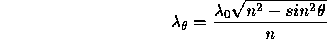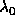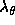Next: Change due to Up: Imaging filters Previous: Calculating of Focus

## Calculation of Central Wavelength

When interference filters are used at angles other than normal incidence, the optical path difference between the direct transmitted beam and the multiple order reflections within the cavity decreases, causing a corresponding decrease in the wavelength of peak transmission. Changes in the bandwidth and transmission characteristics are generally small (for the relatively wide passbands used here).

For angular tilts of less than 30, the central wavelength can be calculated from the expressionwhere= Central wavelength at normal incidence= Central wavelength at the off-normal angle,and n = the refractive index of the interference coatings of the filter.

Tue Aug 15 16:42:46 BST 1995